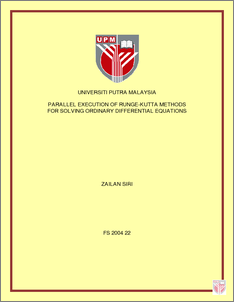# Parallel Execution of Runge-Kutta Methods for Solving Ordinary Differential Equations

## Citation

Siri, Zailan (2004) Parallel Execution of Runge-Kutta Methods for Solving Ordinary Differential Equations. Masters thesis, Universiti Putra Malaysia.

## Abstract

As we know Runge-Kutta method is a one step method hence it is quite limited in terms of implementation in parallel, here we going to exploit and extend the favourable characteristic of Runge-Kutta method so that they can be implemented in parallel. In this thesis we are focusing in two types of Runge-Kutta methods. The first one is the Diagonally Implicit Runge-Kutta (DIRK) method. The method used here is actually have been tailored made for the purpose of parallel machine where the subsequent functions evaluations do not depend on the previous function evaluations. The second family of Runge-Kutta method is the block Runge-Kutta both explicit and implicit. In this study, we exploit these methods so that we can implement in parallel mode. The C programming of the methods employed are run on a shared memory Sequent SE30 parallel computer. All the numerical results are given to illustrate the algorithms developed for the cases that we were tested. The numerical results show that the parallel algorithms of diagonally implicit Runge-Kutta (DIRK), block explicit Runge-Kutta (BERK) and block diagonally implicit Runge-Kutta (BDIRK) methods is better than sequential modes because the parallel execution time is smaller than sequential execution time.Preview
PDF
FS_2004_22.pdfView Item